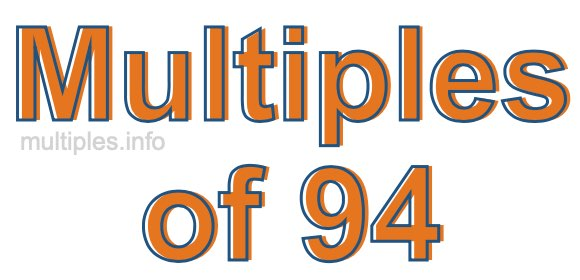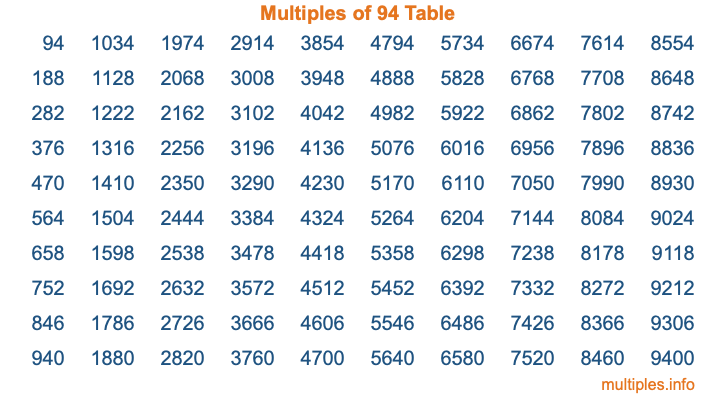Multiples of 94Welcome to the Multiples of 94 page. Here we will first teach you everything you will ever need to know about the multiples of 94, and then give you a study guide summary of everything we taught you to make sure you remember it all. Use this page to look up facts and learn information about the multiples of 94. This page will make you a multiples of ninety-four expert!

Definition of Multiples of 94
Multiples of 94 are all the numbers that when divided by 94 equal an integer. Each of the multiples of 94 are called a multiple. A multiple of 94 is created by multiplying 94 by an integer.

Therefore, to create a list of multiples of 94, you start with 1 multiplied by 94, then 2 multiplied by 94, then 3 multiplied by 94, and so on for as long as you want. Thus, the list of the first five multiples of 94 is 94, 188, 282, 376, and 470. To see a larger list of multiples of 94, see the printable image of Multiples of 94 further down on this page. We also have a category where you can choose any nth multiple of 94.

Multiples of 94 Checker
The Multiples of 94 Checker below checks to see if any number of your choice is a multiple of 94. In other words, it checks to see if there is any number (integer) that when multiplied by 94 will equal your number. To do that, we divide your number by 94. If the the quotient is an integer, then your number is a multiple of 94.

Is  a multiple of 94?

Least Common Multiple of 94 and ...
A Least Common Multiple (LCM) is the lowest multiple that two or more numbers have in common. This is also called the smallest common multiple or lowest common multiple and is useful to know when you are adding our subtracting fractions. Enter one or more numbers below (94 is already entered) to find the LCM.

Check out our LCM Calculator if you need more details about the Least Common Multiple or if you need the LCM for different numbers for adding and subtraction fractions.

nth Multiple of 94
As we stated above, 94 is the first multiple of 94, 188 is the second multiple of 94, 282 is the third multiple of 94, and so on. Enter a number below to find the nth multiple of 94.

th multiple of 94

Multiples of 94 vs Factors of 94
94 is a multiple of 94 and a factor of 94, but that is where the similarities end. All postive multiples of 94 are 94 or greater than 94. All positive factors of 94 are 94 or less than 94.

Below is the beginning list of multiples of 94 and the factors of 94 so you can compare:

Multiples of 94: 94, 188, 282, 376, 470, etc.

Factors of 94: 1, 2, 47, 94

As you can see, the multiples of 94 are all the numbers that you can divide by 94 to get a whole number. The factors of 94, on the other hand, are all the whole numbers that you can multiply by another whole number to get 94.

It's also interesting to note that if a number (x) is a factor of 94, then 94 will also be a multiple of that number (x).

Multiples of 94 vs Divisors of 94
The divisors of 94 are all the integers that 94 can be divided by evenly. Below is a list of the divisors of 94.

Divisors of 94: 1, 2, 47, 94

The interesting thing to note here is that if you take any multiple of 94 and divide it by a divisor of 94, you will see that the quotient is an integer.

Multiples of 94 Table
Below is an image of the first 100 multiples of 94 in a table. The table is in chronological order, column by column. The first column has the first ten multiples of 94, the second column has the next ten multiples of 94, and so on.The Multiples of 94 Table is also referred to as the 94 Times Table or Times Table of 94. You are welcome to print out our table for your studies.

Negative Multiples of 94
Although not often discussed or needed in math, it is worth mentioning that you can make a list of negative multiples of 94 by multiplying 94 by -1, then by -2, then by -3, and so on, to get the following list of negative multiples of 94:

-94, -188, -282, -376, -470, etc.

Multiples of 94 Summary
Below is a summary of important Multiples of 94 facts that we have discussed on this page. To retain the knowledge on this page, we recommend that you read through the summary and explain to yourself or a study partner why they hold true.

There are an infinite number of multiples of 94.

A multiple of 94 divided by 94 will equal a whole number.

94 divided by a factor of 94 equals a divisor of 94.

The nth multiple of 94 is n times 94.

The largest factor of 94 is equal to the first positive multiple of 94.

94 is a multiple of every factor of 94.

94 is a multiple of 94.

A multiple of 94 divided by a divisor of 94 equals an integer.

94 divided by a divisor of 94 equals a factor of 94.

Any integer times 94 will equal a multiple of 94.

Multiples of a Number
Here you can get the multiples of another number, all with the same attention to detail as we did for multiples of 94 on this page.

Multiples of
Multiples of 95
Did you find our page about multiples of ninety-four educational? Do you want more knowledge? Check out the multiples of the next number on our list!

Copyright  |   Privacy Policy  |   Disclaimer  |   Contact